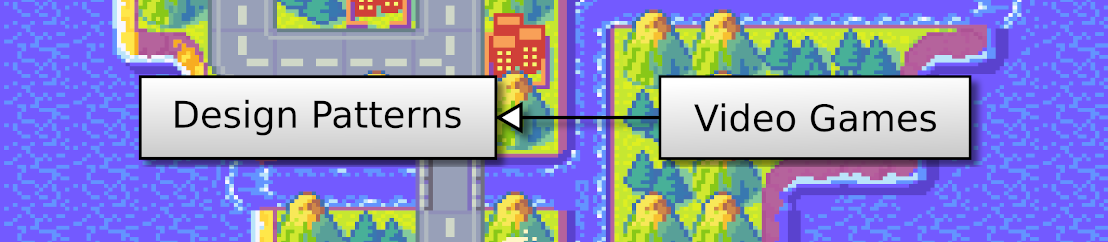## OpenGL 2D Facade (29): Z-Buffer and point light

In the previous post, I added light around the main character. In this post, I improve it with the OpenGL Z-Buffer. For instance, I no more light a tree when the hero is behind it. I also add light attenuation based on the distance to the hero.

This post is part of the OpenGL 2D Facade series

## Objective

As you can see in this video, there is no more light on a tree when the main character is behind it:

## Light and Z-Buffer

In the previous post, we created this fragment shader:

``````#version 330
in vec2 outputUV;
in vec4 worldVertex;
out vec4 color;
uniform sampler2D textureColors;
uniform vec4 colorFactors;
uniform vec2 pixelSize;
uniform vec3 lightProperties;
void main() {
// Color from tileset
color = texture(textureColors, outputUV);

// Compute distance to light
float worldLocationX = (worldVertex.x + 1) / pixelSize.x;
float worldLocationY = -(worldVertex.y - 1) / pixelSize.y;
float diffX = worldLocationX - lightProperties.x;
float diffY = worldLocationY - lightProperties.y;
float dist = sqrt(diffX * diffX + diffY * diffY);

// Apply color factors if far from light
color *= colorFactors;
}
} ``````

We apply the light effect in lines 22-24: if the distance between the light location and the pixel is larger than the radius of the point light, we change the color using factors. These factors reduce the intensity of red, green, and blue channels. With factors like (0.8, 0.6, 0.4), we got a night effect.

We add a second condition to allow the darkening of the pixel when the light is in front of it:

``````float lightRadius = lightProperties.w;
if (dist >= lightRadius || lightProperties.z > worldVertex.z) {
color *= colorFactors;
}``````

The `z` attribute of `lightProperties` contains the depth of the light. We set it outside the fragment, for instance we can choose the depth of the main character.

The `z` attribute of `worldVertex' contains the depth of the pixel the fragment shader is processing. It is a value we set when we set tiles/mesh faces.

## Tiles/mesh faces depth

### Remindings

In this post, we define the depth of tiles for each layer type.

For most layers, we compute a depth for each row of the grid, for instance:

• Layer 0 Row 1: depth 1023
• Layer 0 Row 2: depth 1013
• Layer 0 Row 3: depth 1003
• etc.

We use the same depth for a row because we assume that there is no tile overlapping on the left or the right (otherwise, we need a depth value for each tile).

Note that there is a step large enough to interleave depth values of other layers. For instance, the second layer in front of the first one can get these values:

• Layer 1 Row 1: depth 1022
• Layer 1 Row 2: depth 1012
• Layer 1 Row 3: depth 1002
• etc.

Using this trick, we can draw the first layer entirely before the second one. Thus, it speeds up the rendering while leading to the expected rendering.

### Big tiles

The light effect does not work with this definition of tile depths: there is no light in tiles below the character:

We need to change the depth of tiles that should be behind the light. There is no single rule; here, I propose to consider that big tiles are always in front and the others behind.

In the `setTile()` method of the `OpenGLGridLayer` class, we update the depth according to the height of the tile:

``````def setTile(self, x: int, y: int, tileX: int, tileY: int, width: int = 1, height: int = 1):
faceIndex = x + y * self.__width
self.setFaceTileLocation(faceIndex, x, y, width, height)
self.setFaceTileTexture(faceIndex, tileX, tileY, width, height)
if height == 1:
self.setFaceDepth(faceIndex, self.__depths)
else:
self.setFaceDepth(faceIndex, self.__depths[y])``````

The `depths` attribute contains the depth values for each grid row (we compute it in the `updateDepths()` method). When the tile is small (height is 1), we set the depth of the first row, always behind all other rows. Since we interleave row depths in layers, these tiles are always behind tiles of the following layers. In the other case, we set the depth as before.

## Light attenuation

We implement distance-based light attenuation in the fragment shader when the current pixel is enlighted (lines 5-8):

``````float lightRadius = lightProperties.w;
if (dist >= lightRadius || lightProperties.z > worldVertex.z) {
color *= colorFactors;
}
else {
float f = dist / lightRadius;
color *= 1.0f - (1.0f - colorFactors) * f * f;
}``````

Line 6 compares the distance to the light location with its radius. It always leads to a value between 0 and 1; 0 when we are far from the light, and 1 when on it.

Line 7 updates the colors according to `f`. We use a quadratic expression `f * f` to get a non-linear attenuation. We could also use other functions like exponential or logarithm, depending on the effect we want to get.